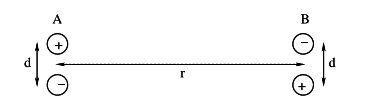# Electric Dipole

## Homework Statement

An electric dipole consists of two charges of equal magnitude ##q## and opposite sign, which are kept at a distance ##d## apart. The dipole moment is ##p= qd## .
Let us next place two such dipoles, placed at distance ##r## apart, as shown in the accompanying figure.a) Assuming that the potential energy for the charges while at infinity is zero, find the exact potential energy of the configuration in terms of ##d, r, q ## and fundamental constants.

b)When ##d<<r## , approximate your previous result in terms of ##p, r ## and fundamental constants.

## Homework Equations

##V = \frac {1}{4\pi \epsilon_0} \frac{q}{r}##
##W = Vq##

## The Attempt at a Solution

For the positve charge of A dipole, I calculated the potential energy
##= +q \cdot \frac{1}{4\pi \epsilon _0} (\frac{-q}{r} + \frac {+q}{\sqrt{r^2 +d^2}} + \frac{-q}{d}) ##, because, ##W = Vq##
The potental energy for the other three charges are the same.
So, the potential energy of the configuration is
## = 4q \cdot \frac{1}{4\pi \epsilon _0} (\frac{-q}{r} + \frac {+q}{\sqrt{r^2 +d^2}} + \frac{-q}{d})##
##= \frac{q^2}{\pi \epsilon _0} (- \frac{1}{r} + \frac {1}{\sqrt{r^2 +d^2}} - \frac{1}{d})##;

Then , I can't find any way how to approximate the result when ##d << r##;
In this case, I substituted ##r^2## for ##r^2 + d^2## ;
So, two of the terms in bracket are cancelled.
Then I plugged in ## q = \frac{p}{d}## ;
But, still ##d## is there.

Orodruin
Staff Emeritus
Homework Helper
Gold Member
2021 Award
For the positve charge of A dipole, I calculated the potential energy
##= +q \cdot \frac{1}{4\pi \epsilon _0} (\frac{-q}{r} + \frac {+q}{\sqrt{r^2 +d^2}} + \frac{-q}{d}) ##, because, ##W = Vq##
The potental energy for the other three charges are the same.

This is not correct. The potential energy (q1q2/4πεr) is for a pair of charges separated by a given distance. The way you are implementing it, you are double counting each pair and end up with double the potential energy.

So, the potential energy of the configuration is
## = 4q \cdot \frac{1}{4\pi \epsilon _0} (\frac{-q}{r} + \frac {+q}{\sqrt{r^2 +d^2}} + \frac{-q}{d})##
##= \frac{q^2}{\pi \epsilon _0} (- \frac{1}{r} + \frac {1}{\sqrt{r^2 +d^2}} - \frac{1}{d})##;

Then , I can't find any way how to approximate the result when ##d << r##;
In this case, I substituted ##r^2## for ##r^2 + d^2## ;
So, two of the terms in bracket are cancelled.
Then I plugged in ## q = \frac{p}{d}## ;
But, still ##d## is there.

The term proportional to 1/d in your parenthesis is the energy from the construction of the dipoles themselves. This is generally going to be much higher than the potential between the dipoles. Just substituting ##r^2## for ##r^2 + d^2## is going to make the potential between the dipoles disappear (as you noticed, the terms cancelled) and you will end up with an uninteresting result. Instead, you should make an expansion of the square root for small ##d##.

This is not correct. The potential energy (q1q2/4πεr) is for a pair of charges separated by a given distance. The way you are implementing it, you are double counting each pair and end up with double the potential energy.
I agree with you. So, the potential energy will be half of my calculation.
The term proportional to 1/d in your parenthesis is the energy from the construction of the dipoles themselves. This is generally going to be much higher than the potential between the dipoles. Just substituting r2r^2 for r2+d2r^2 + d^2 is going to make the potential between the dipoles disappear (as you noticed, the terms cancelled) and you will end up with an uninteresting result. Instead, you should make an expansion of the square root for small dd.
So, I need to leave the term ##\frac {1}{d}## ?
Then it becomes,
##\frac{1}{2} \cdot \frac{q^2}{\pi \epsilon _0}(- \frac{1}{r} + \frac{1}{\sqrt{d^2 + r^2}})##
##= \frac{q^2}{2 \pi \epsilon _0}(- \frac{1}{r} + \frac{1}{r} \cdot [1 + (\frac{d}{r})^2]^{- \frac{1}{2}})##
##= \frac{q^2}{2 \pi \epsilon _0} \frac{1}{r} (-1+ [1 - \frac{1}{2}(\frac{d}{r})^2] )##
##= \frac{q^2}{2 \pi \epsilon _0} \frac{1}{r} (- \frac{1}{2}(\frac{d}{r})^2))##
##= - \frac{p^2}{4 \pi \epsilon _0 r^3} ##

Last edited:
Orodruin
Staff Emeritus
$$\frac{q^2}{2 \pi \epsilon _0 r}\left(- 1 + \frac{1}{ \sqrt{1 + (\frac{d}{r})^2}}\right)$$